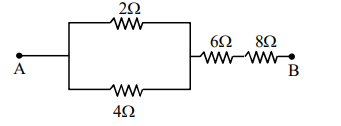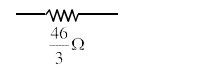# If you are provided a set of resistances`
Question:

If you are provided a set of resistances $2 \Omega, 4 \Omega$, $6 \Omega$ and $8 \Omega$. Connect these resistances so as to obtain an equivalent resistance of $\frac{46}{3} \Omega$.

1. $4 \Omega$ and $6 \Omega$ are in parallel with $2 \Omega$ and $8 \Omega$ in series

2. $6 \Omega$ and $8 \Omega$ are in parallel with $2 \Omega$ and $4 \Omega$ in series

3. $2 \Omega$ and $6 \Omega$ are in parallel with $4 \Omega$ and $8 \Omega$ in series

4. $2 \Omega$ and $4 \Omega$ are in parallel with $6 \Omega$ and $8 \Omega$ in series

Correct Option: , 4

Solution:Ans 4